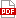Datasheet下载
• 制造商： Analog
• 库位|库存： xxxx|xxxx
• 要求：
 数量阶梯 香港交货 国内含税 +xxxx \$xxxx ￥xxxx

 参数 数值 产品目录 集成电路 (IC)半导体 描述 IC I/O PORT 8BIT ANALOG 24-SOIC模数转换器 - ADC 8-BIT CMOS I/O PORT w/ SGL Output 产品分类 接口 - 专用 品牌 Analog Devices 产品手册 点击此处下载产品Datasheet 产品图片rohs 符合RoHS无铅 / 符合限制有害物质指令(RoHS)规范要求 产品系列 数据转换器IC,模数转换器 - ADC,Analog Devices AD7569JRZLC²MOS 数据手册 点击此处下载产品Datasheet 产品型号 AD7569JRZ 产品目录页面 点击此处下载产品Datasheet 产品种类 模数转换器 - ADC 供应商器件封装 24-SOIC W 信噪比 44 dB 分辨率 8 bit 包装 管件 商标 Analog Devices 安装类型 表面贴装 安装风格 SMD/SMT 封装 Tube 封装/外壳 24-SOIC（0.295"，7.50mm 宽） 封装/箱体 SOIC-24 工作电源电压 5 V 工厂包装数量 31 应用 模拟 I/O 接口 总线 接口类型 Parallel 最大功率耗散 85 mW 最大工作温度 + 125 C 最小工作温度 - 55 C 标准包装 31 电压-电源 4.75 V ~ 5.25 V 电压参考 Internal 系列 AD7569 结构 SAR 转换器数量 1 转换速率 500 kS/s 输入类型 Single-Ended 通道数量 1 Channel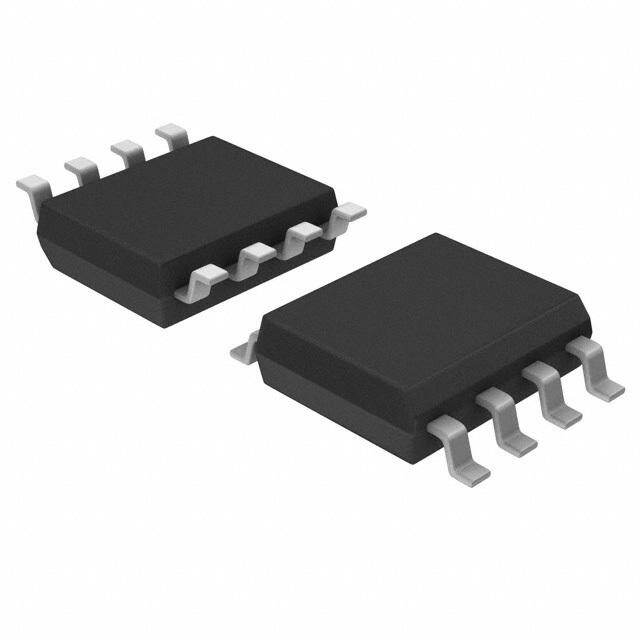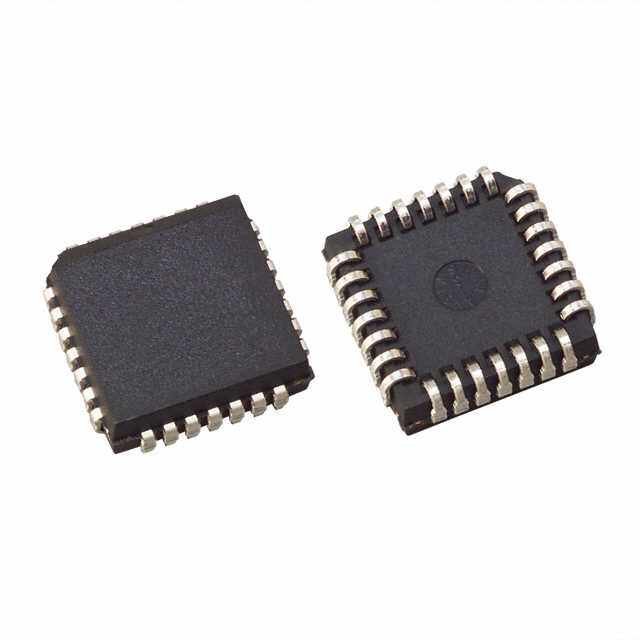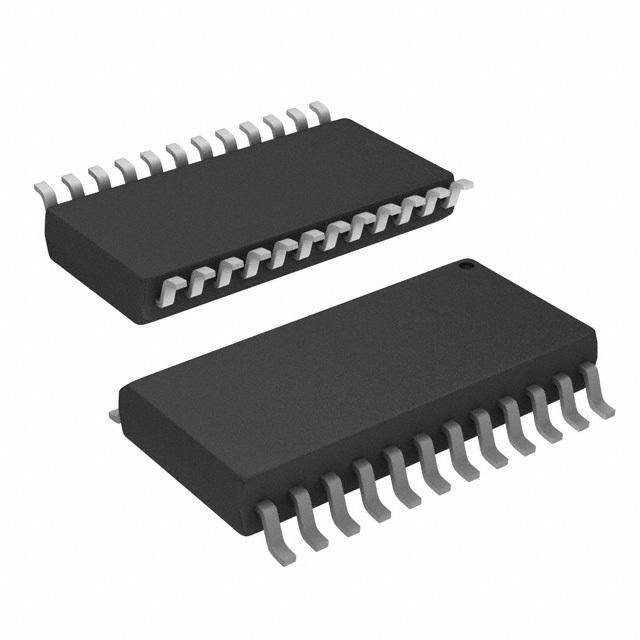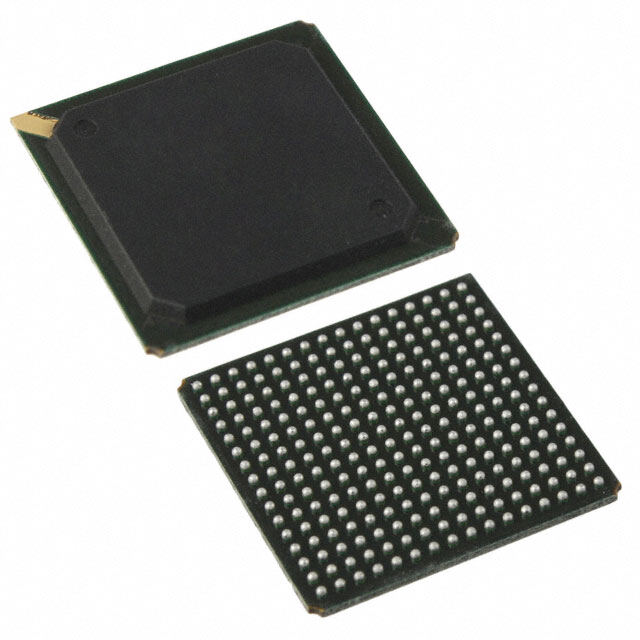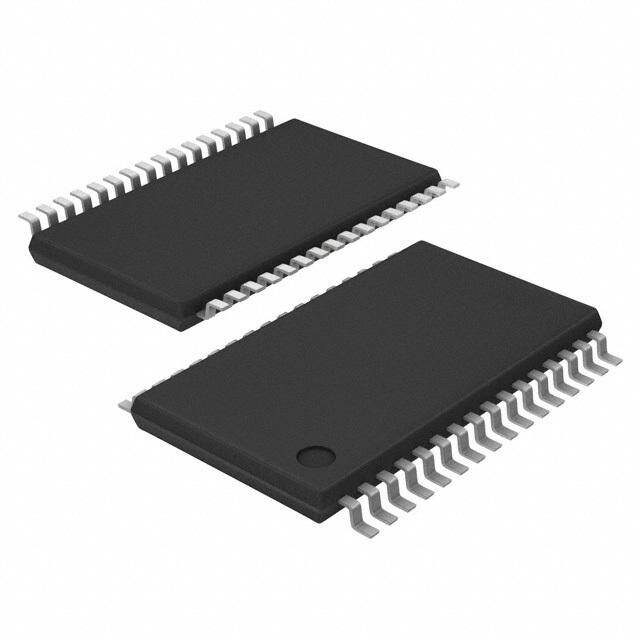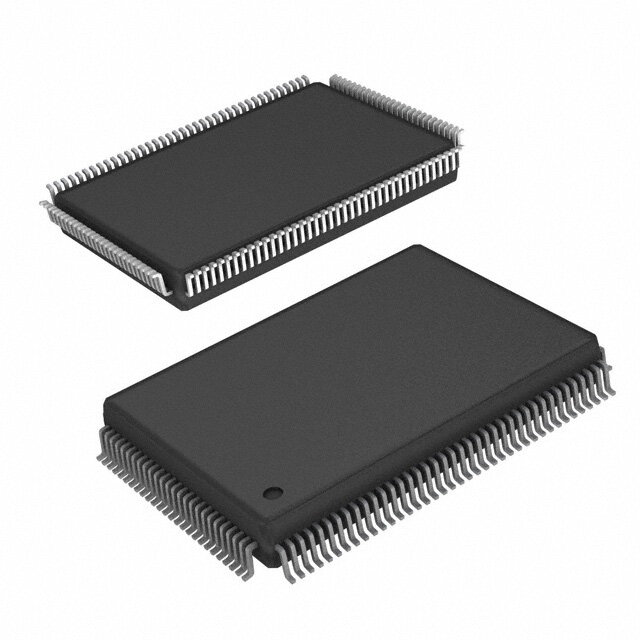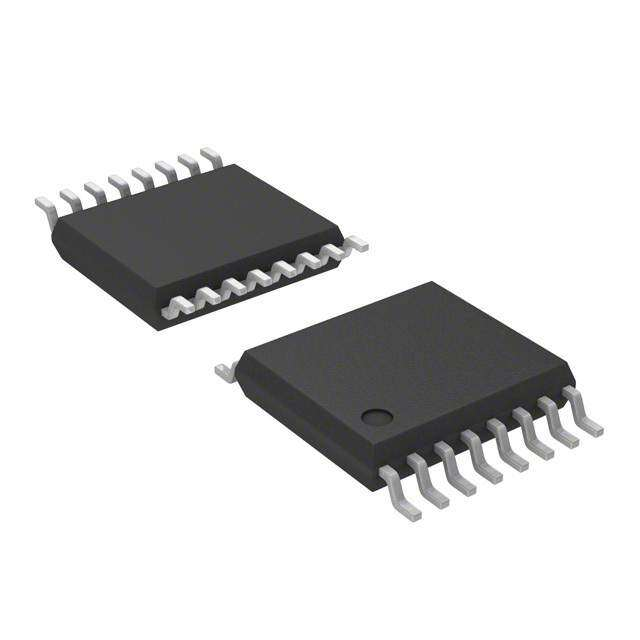STLED316SMTR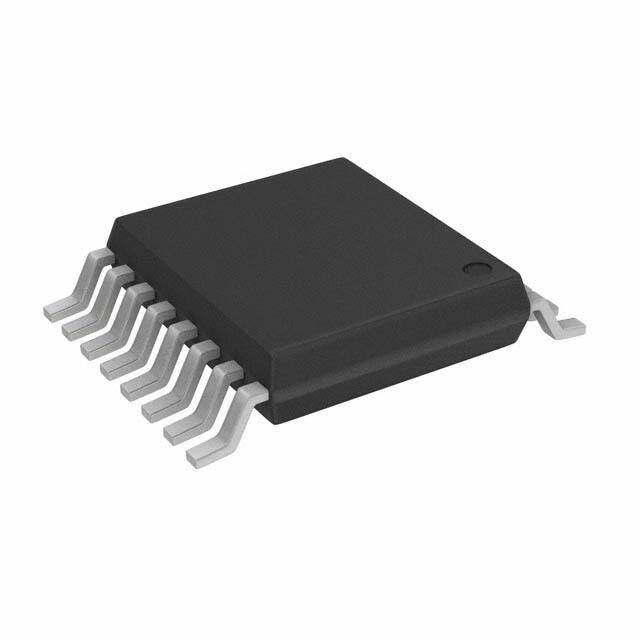PCA9546APW,112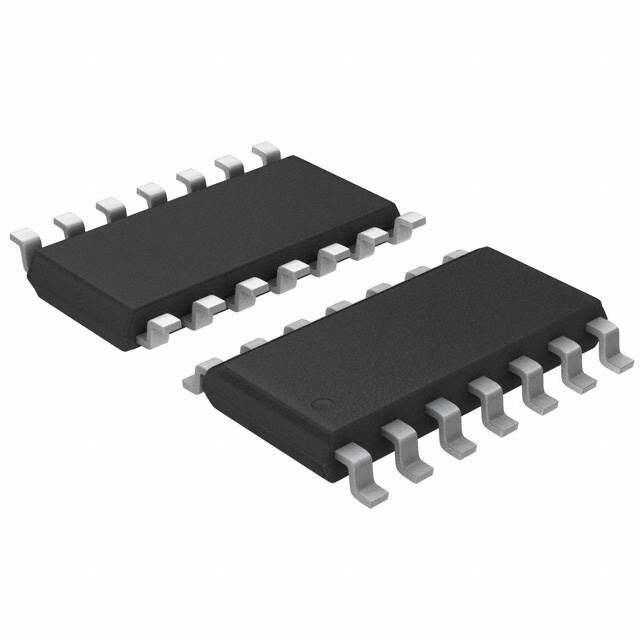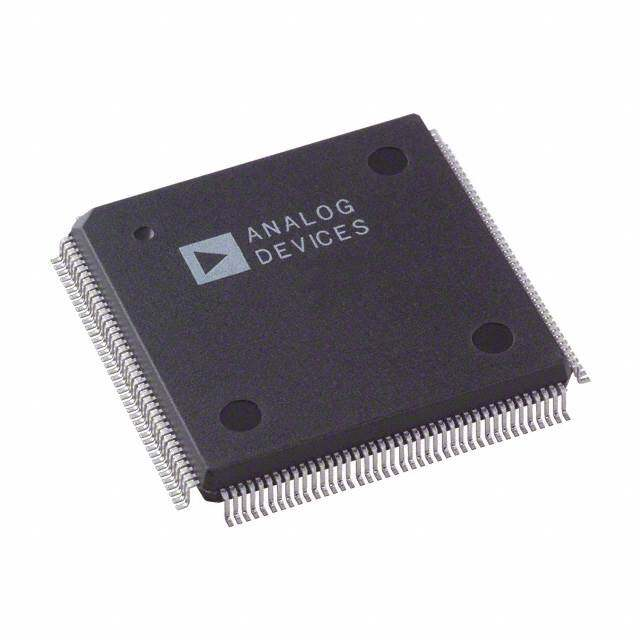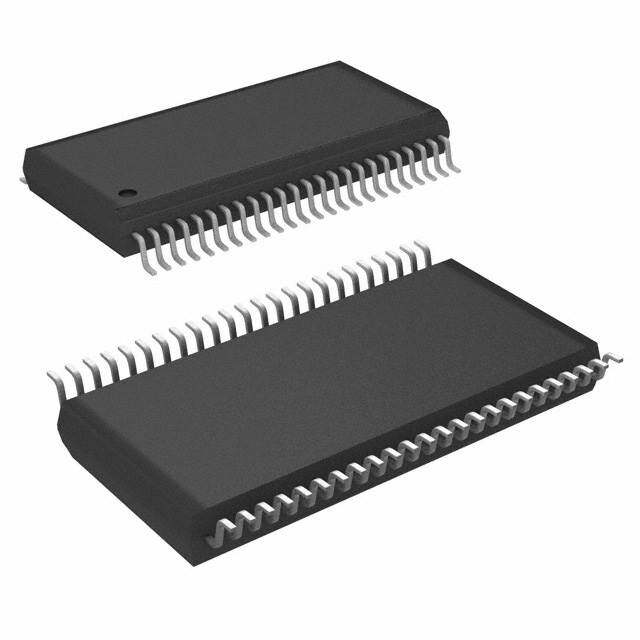DS90CF366MTD/NOPB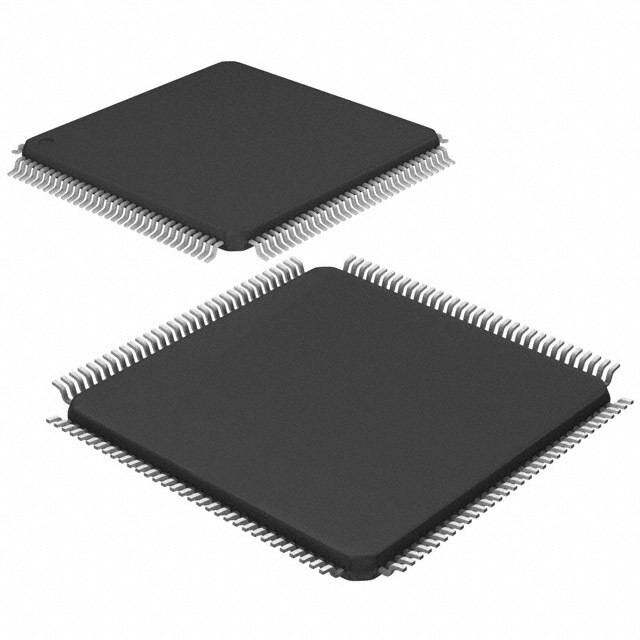MEC1308-NU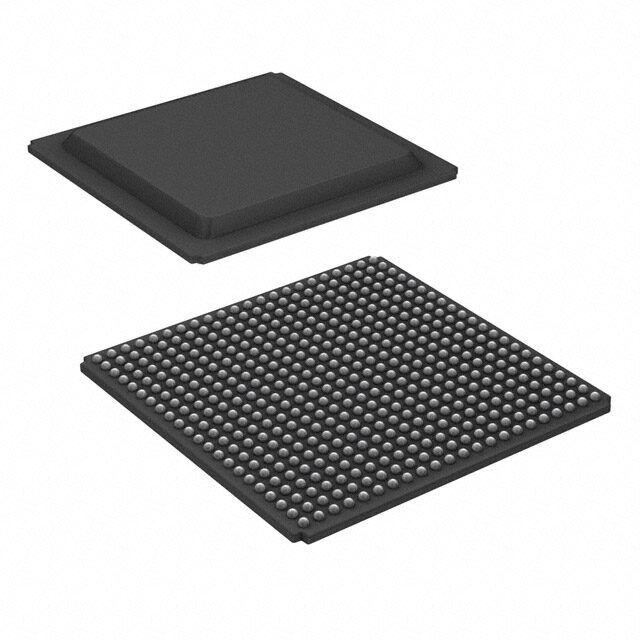89H32NT24AG2ZCHLG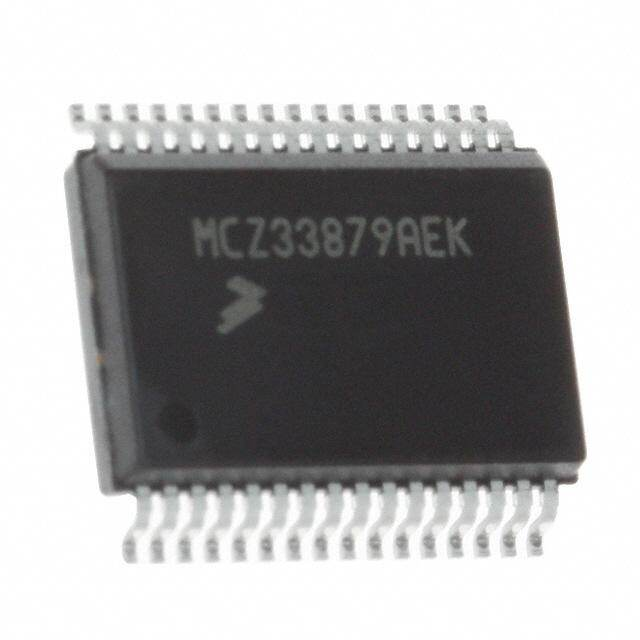MC33972ATEKR2

Analog

### PDF Datasheet 数据手册内容提取

AD7569/AD7669 ADC SPECIFICATIONS (V = +5 V (cid:54) 5%; V 1 = RANGE = AGND = AGND = DGND = 0 V; f = 5 MHz external unless other- DD SS DAC DAC CLK wise noted. All specifications T to T unless otherwise noted.) Specifications apply to Mode 1 interface. MIN MAX AD7569 J, A Versions3 AD7569 AD7669 K, B AD7569 AD7569 Parameter J Version Versions S Version T Version Units Conditions/Comments DC ACCURACY Resolution3 8 8 8 8 Bits Total Unadjusted Error4 – 3 – 3 – 4 – 4 LSB typ Relative Accuracy4 – 1 – 1/2 – 1 – 1/2 LSB max Differential Nonlinearity4 – 1 – 3/4 – 1 – 3/4 LSB max No Missing Codes Unipolar Offset Error Typical tempco is 10 m V/(cid:176)C for +1.25 V range; VSS = 0 V @ +25(cid:176)C – 2 – 1.5 – 2 – 1.5 LSB max TMIN to TMAX – 3 – 2.5 – 3 – 2.5 LSB max Bipolar Zero Offset Error Typical tempco is 20 m V/(cid:176)C for + 1.25 V range; VSS = –5 V @ +25(cid:176)C – 3 – 2.5 – 3 – 2.5 LSB max TMIN to TMAX – 3.5 – 3 – 4 – 3.5 LSB max Full-Scale Error5 VDD = 5 V @ +25(cid:176)C –4, +0 –4, +0 –4, +0 –4, +0 LSB max TMIN to TMAX –5.5, +1.5 –5.5, +1.5 –7.5, +2 –7.5, +2 LSB max D Full Scale/D VDD, TA = +25(cid:176)C 0.5 0.5 0.5 0.5 LSB max VIN = +2.5 V; D VDD = – 5% D Full Scale/D VSS, TA = +25(cid:176)C 0.5 0.5 0.5 0.5 LSB max VIN = –2.5 V; D VSS = – 5% DYNAMIC PERFORMANCE Signal-to-Noise Ratio4 (SNR) 44 46 44 45 dB min VIN = 100 kHz full-scale sine wave with fSAMPLING = 400 kHz6 Total Harmonic Distortion4 (THD) 48 48 48 48 dB max VIN = 100 kHz full-scale sine wave with fSAMPLING = 400 kHz6 Intermodulation Distortion4 (IMD) 60 60 60 60 dB typ fa = 99 kHz, fb = 96.7 kHz with fSAMPLING = 400 kHz Frequency Response 0.1 0.1 0.1 0.1 dB typ VIN = – 2.5 V, dc to 200 kHz sine wave Track/Hold Acquisition Time7 200 200 300 300 ns typ ANALOG INPUT Input Voltage Ranges Unipolar 0 to +1.25/ +2.5 Volts VDD = +5 V; VSS = 0 V Bipolar – 1.25/– 2.5 Volts VDD = +5 V; VSS = –5 V Input Current – 300 – 300 – 300 – 300 m A max See equivalent circuit Figure 5 Input Capacitance 10 10 10 10 pF typ LOGIC INPUTS CS, RD, ST, CLK, RESET, RANGE Input Low Voltage, VINL 0.8 0.8 0.8 0.8 V max Input High Voltage, VINH 2.4 2.4 2.4 2.4 V min Input Capacitance8 10 10 10 10 pF max CS, RD, ST, RANGE, RESET Input Leakage Current 10 10 10 10 m A max V IN = 0 to VDD CLK Input Current IINL –1.6 –1.6 –1.6 –1.6 mA max VIN = 0 V IINH 40 40 40 40 m A max VIN = VDD LOGIC OUTPUTS DB0–DB7, INT, BUSY VOL, Output Low Voltage 0.4 0.4 0.4 0.4 V max ISINK = 1.6 mA VOH, Output High Voltage 4.0 4.0 4.0 4.0 V min ISOURCE = 200 m A DB0–DB7 Floating State Leakage Current 10 10 10 10 m A max Floating State Output Capacitance8 10 10 10 10 pF max Output Coding (Single Supply) Binary Output Coding (Dual Supply) 2s Complement CONVERSION TIME With External Clock 2 2 2 2 m s max fCLK = 5 MHz With Internal Clock, TA = +25(cid:176)C 1.6 1.6 1.6 1.6 m s min Using recommended clock components shown in Figure 21. 2.6 2.6 2.6 2.6 m s max Clock frequency can be adjusted by varying RCLK. POWER REQUIREMENTS As per DAC Specifications NOTES 1Except where noted, specifications apply for all ranges including bipolar ranges with dual supply operation. 2Temperature ranges are as follows:J, K versions; 0(cid:176)C to +70(cid:176)C A, B versions; –40(cid:176)C to +85(cid:176)C S, T versions; –55(cid:176)C to +125(cid:176)C 31 LSB = 4.88 mV for 0 V to +1.25 V range, 9.76 mV for 0 V to +2.5 V and – 1.25 V ranges and 19.5 mV for +2.5 V range. 4See Terminology. 5Includes internal voltage reference error and is calculated after offset error has been adjusted out. Ideal unipolar last code transition occurs at (FS – 3/2 LSB). Ideal bipolar last code transition occurs at (FS/2 – 3/2 LSB). 6Exact frequencies are 101 kHz and 384 kHz to avoid harmonics coinciding with sampling frequency. 7Rising edge of BUSY to falling edge of ST. The time given refers to the acquisition time, which gives a 3 dB degradation in SNR from the tested figure. 8Sample tested at +25(cid:176)C to ensure compliance. Specifications subject to change without notice. REV. B –3–

AD7569/AD7669–TIMING CHARACTERISTICS1 (See Figures 8, 10, 12; V = 5 V (cid:54) 5%; V = 0 V or –5 V (cid:54) 5%) DD SS Limit at Limit at Limit at T , T T , T MIN MAX MIN MAX Parameter 25(cid:56)C (All Grades) (J, K, A, B Grades) (S, T Grades) Units Test Conditions/Comments DAC Timing t 80 80 90 ns min WR Pulse Width 1 t 0 0 0 ns min CS, A/B to WR Setup Time 2 t 0 0 0 ns min CS, A/B to WR Hold Time 3 t 60 70 80 ns min Data Valid to WR Setup Time 4 t 10 10 10 ns min Data Valid to WR Hold Time 5 ADC Timing t 50 50 50 ns min ST Pulse Width 6 t 110 130 150 ns max ST to BUSY Delay 7 t 20 30 30 ns max BUSY to INT Delay 8 t 0 0 0 ns min BUSY to CS Delay 9 t 0 0 0 ns min CS to RD Setup Time 10 t 60 75 90 ns min RD Pulse Width Determined by t . 11 13 t 0 0 0 ns min CS to RD Hold Time 12 t 2 60 75 90 ns max Data Access Time after RD; C = 20 pF 13 L 95 120 135 ns max Data Access Time after RD; C = 100 pF L t 3 10 10 10 ns min Bus Relinquish Time after RD 14 60 75 85 ns max t 65 75 85 ns max RD to INT Delay 15 t 120 140 160 ns max RD to BUSY Delay 16 t 2 60 75 90 ns max Data Valid Time after BUSY; C = 20 pF 17 L 90 115 135 ns max Data Valid Time after BUSY; C = 100 pF L NOTES 1Sample tested at +25(cid:176)C to ensure compliance. All input control signals are specified with t = t = 5 ns (10% to 90% of +5 V) and timed from a voltage level of 1.6 V. R F 2t and t are measured with the load circuits of Figure 1 and defined as the time required for an output to cross either 0.8 V or 2.4 V. 13 17 3t is defined as the time required for the data line to change 0.5 V when loaded with the circuit of Figure 2. l4 Specifications subject to change without notice. a. High-Z to V b. High-Z to V a. V to High-Z b. V to High-Z OH OL OH OL Figure 1.Load Circuits for Data Access Time Test Figure 2.Load Circuits for Bus Relinquish Time Test ABSOLUTE MAXIMUM RATINGS V to AGND or AGND . . . . . . . . . . . . .–0.3 V, +7 V Power Dissipation (Any Package) to +75(cid:176) C . . . . . . . .450 mW VDD to DGNDD .A C. . . . . . . . . .A .D .C . . . . . . . . . . . . . .–0.3 V, +7 V Derates above 75(cid:176) C by . . . . . . . . . . . . . . . . . . . . . 6 mW/(cid:176) C DD Operating Temperature Range V to V . . . . . . . . . . . . . . . . . . . . . . . . . . . . .–0.3 V, +14 V DD SS Commercial (J, K) . . . . . . . . . . . . . . . . . . . . . .0(cid:176) C to +70(cid:176) C AGND or AGND to DGND . . . .–0.3 V, V + 0.3 V AGNDDAC to AGNDADC . . . . . . . . . . . . . . . . . . . . .D .D . . .– 5 V Industrial (A, B) . . . . . . . . . . . . . . . . . . . . . –40(cid:176) C to +85(cid:176) C DAC ADC Extended (S, T) . . . . . . . . . . . . . . . . . . . . –55(cid:176) C to +125(cid:176) C Logic Voltage to DGND . . . . . . . . . . . . .–0.3 V, V + 0.3 V DD Storage Temperature Range . . . . . . . . . . . . –65(cid:176) C to +150(cid:176) C CLK Input Voltage to DGND . . . . . . . . .–0.3 V, V + 0.3 V DD Lead Temperature (Soldering, 10 secs) . . . . . . . . . . . +300(cid:176) C V (V A, V B) to OUT OUT OUT AGND1 . . . . . . . . . . . . . . . . . V – 0.3 V, V + 0.3 V *Stresses above those listed under “Absolute Maximum Ratings” may cause DAC SS DD V to AGND . . . . . . . . . . . . . . . V – 0.3 V, V + 0.3 V permanent damage to the device. This is a stress rating only; functional operation IN ADC SS DD of the device at these or any other condition above those indicated in the NOTE operational sections of this specification is not implied. Exposure to absolute 1Output may be shorted to any voltage in the range V to V provided that the SS DD maximum rating conditions for extended periods may affect device reliability. power dissipation of the package is not exceeded. Typical short circuit current for a short to AGND or V is 50 mA. SS CAUTION ESD (electrostatic discharge) sensitive device. Electrostatic charges as high as 4000V readily WARNING! accumulate on the human body and test equipment and can discharge without detection. Although the AD7569/AD7669 features proprietary ESD protection circuitry, permanent dam- age may occur on devices subjected to high energy electrostatic discharges. Therefore, proper ESD precautions are recommended to avoid performance degradation or loss of functionality. ESD SENSITIVE DEVICE –4– REV. B

AD7569/AD7669 NOTE: Digital Feedthrough The term DAC (Digital-to-Analog Converter) throughout the Digital Feedthrough is also a measure of the impulse injected to data sheet applies equally to the dual DACs in the AD7669 as the analog output from the digital inputs, but is measured when well as to the single DAC of the AD7569 unless otherwise the DAC is not selected. It is essentially feedthrough across the stated. It follows that the term V applies to both V A and die and package. It is also a measure of the glitch impulse trans- OUT OUT V B of the AD7669 also. ferred to the analog output when data is read from the internal OUT ADC. It is specified in nV secs and is measured with WR high TERMINOLOGY and a digital code change from all 0s to all 1s. Total Unadjusted Error Total unadjusted error is a comprehensive specification that in- DAC-to-DAC Crosstalk (AD7669 Only) cludes internal voltage reference error, relative accuracy, gain The glitch energy transferred to the output of one DAC due to and offset errors. an update at the output of the second DAC. The figure given is the worst case and is expressed in nV secs. It is measured with Relative Accuracy (DAC) an update voltage of full scale. Relative Accuracy or endpoint nonlinearity is a measure of the maximum deviation from a straight line passing through the DAC-to-DAC Isolation (AD7669 Only) endpoints of the DAC transfer function. It is measured after al- DAC-to-DAC Isolation is the proportion of a digitized sine lowing for offset and gain errors. For the bipolar output ranges, wave from the output of one DAC, which appears at the output the endpoints of the DAC transfer function are defined as those of the second DAC (loaded with all 1s). The figure given is the voltages that correspond to negative full-scale and positive full- worst case for the second DAC output and is expressed as a ra- scale codes. For the unipolar output ranges, the endpoints are tio in dBs. It is measured with a digitized sine wave (f = SAMPLING code 1 and code 255. Code 1 is chosen because the amplifier is 100 kHz) of 20 kHz at 2.5 V pk-pk. now working in single supply and, in cases where the true offset Signal-to-Noise Ratio of the amplifier is negative, it cannot be seen at code 0. If the Signal-to-Noise Ratio (SNR) is the measured signal to noise at relative accuracy were calculated between code 0 and code 255, the output of the converter. The signal is the rms magnitude of the “negative offset” would appear as a linearity error. If the off- the fundamental. Noise is the rms sum of all the nonfundamen- set is negative and less than 1 LSB, it will appear at code 1, and tal signals (excluding dc) up to half the sampling frequency. hence the true linearity of the converter is seen between code 1 SNR is dependent on the number of quantization levels used in and code 255. the digitization process; the more levels, the smaller the quanti- Relative Accuracy (ADC) zation noise. The theoretical SNR for a sine wave is given by Relative Accuracy is the deviation of the ADC’s actual code SNR = (6.02N + 1.76) dB transition points from a straight line drawn between the end- where N is the number of bits. Thus for an ideal 8-bit converter, points of the ADC transfer function. For the bipolar input SNR = 50 dB. ranges, these points are the measured, negative, full-scale transi- Harmonic Distortion tion point and the measured, positive, full-scale transition point. Harmonic Distortion is the ratio of the rms sum of harmonics to For the unipolar ranges, the straight line is drawn between the the fundamental. For the AD7569/AD7669, Total Harmonic measured first LSB transition point and the measured full-scale Distortion (THD) is defined as transition point. Differential Nonlinearity (cid:86) (cid:50)+(cid:86) (cid:50)+(cid:86) (cid:50)+(cid:86) (cid:50)+(cid:86) (cid:50) Differential Nonlinearity is the difference between the measured (cid:50)(cid:48)(cid:108)(cid:111)(cid:103) (cid:50) (cid:51) (cid:52) (cid:53) (cid:54) (cid:86) change and an ideal 1 LSB change between any two adjacent (cid:49) codes. A specified differential nonlinearity of – 1 LSB max en- where V1 is the rms amplitude of the fundamental and V2, V3, V , V and V are the rms amplitudes of the individual sures monotonicity (DAC) or no missed codes (ADC). A differ- 4 5 6 ential nonlinearity of – 3/4 LSB max ensures that the minimum harmonics. step size (DAC) or code width (ADC) is 1/4 LSB, and the maxi- Intermodulation Distortion mum step size or code width is 3/4 LSB. With inputs consisting of sine waves at two frequencies, fa and fb, any active device with nonlinearities will create distortion Digital-to-Analog Glitch Impulse products, of order (m + n), at sum and difference frequencies of Digital-to-Analog Glitch Impulse is the impulse injected into the mfa – nfb where m, n = 0, l, 2, 3,… . Intermodulation terms analog output when the digital inputs change state with the are those for which m or n is not equal to zero. For example, DAC selected. It is normally specified as the area of the glitch in the second order terms include (fa + fb) and (fa – fb) and the nV secs and is measured when the digital input code is changed third order terms include (2fa + fb), (2fa – fb), (fa + 2fb) and by 1 LSB at the major carry transition. (fa – 2fb). REV. B –5–

AD7569/AD7669—Typical Performance Graphs Noise Spectral Density vs. Frequency Power Supply Rejection Ratio vs. Frequency Positive-Going Settling Time (– 2.5 V Range) Negative-Going Settling Time (– 2.5 V Range) DAC/ADC Full-Scale Temperature Coefficient IMD Plot for ADC –8– REV. B

AD7569/AD7669 and harmonic distortion performance. Similarly, for inter- modulation distortion, an input (either to V or DAC code) IN consisting of pure sine waves at two frequencies is applied to the AD7569/AD7669. Figure 15.DAC Output Spectrum HISTOGRAM PLOT When a sine wave of specified frequency is applied to the V in- IN put of the AD7569/AD7669 and several thousand samples are taken, it is possible to plot a histogram showing the frequency of occurrence of each of the 256 ADC codes. If a particular step is wider than the ideal 1 LSB width, the code associated with that step will accumulate more counts than for the code for an ideal step. Likewise, a step narrower than ideal width will have fewer Figure 13.ADC FFT Plot counts. Missing codes are easily seen because a missing code means zero counts for a particular code. The absence of large Figure 13 shows a 2048 point FFT plot of the ADC with an in- spikes in the plot indicates small differential nonlinearity. put signal of 130 kHz. The SNR is 48.4 dB. It can be seen that most of the harmonics are buried in the noise floor. It should be Figure 16 shows a histogram plot for the ADC indicating very noted that the harmonics are taken into account when calculat- small differential nonlinearity and no missing codes for an input ing the SNR. The relationship between SNR and resolution (N) frequency of 204 kHz. For a sine-wave input, a perfect ADC is expressed by the following equation: would produce a cusp probability density function described by the equation SNR = (6.02N + 1.76) dB (cid:49) (cid:112)(cid:40)(cid:86)(cid:41)= This is for an ideal part with no differential or integral linearity p (cid:40)(cid:65)(cid:50)- (cid:86)(cid:50)(cid:41)(cid:49)(cid:47)(cid:50) errors. These errors will cause a degradation in SNR. By work- ing backward from the above equation, it is possible to get a where A is the peak amplitude of the sine wave and p(V) the measure of ADC performance expressed in effective number of probability of occurrence at a voltage V. bits (N). This effective number of bits is plotted versus fre- The histogram plot of Figure 16 corresponds very well with this quency in Figure 14. The effective number of bits typically falls cusp shape. between 7.7 and 7.8, corresponding to SNR figures of 48.1 dB Further typical plots of the performance of the AD7569/AD7669 and 48.7 dB. are shown in the Typical Performance Graphs section of the data Figure 15 shows a spectrum analyzer plot of the output spec- sheet. trum from the DAC with an ideal sine-wave table loaded to the data inputs of the DAC. In this case, the SNR is 46 dB. Figure 14.Effective Number of Bits vs. Frequency Figure 16.ADC Histogram Plot REV. B –13–